Question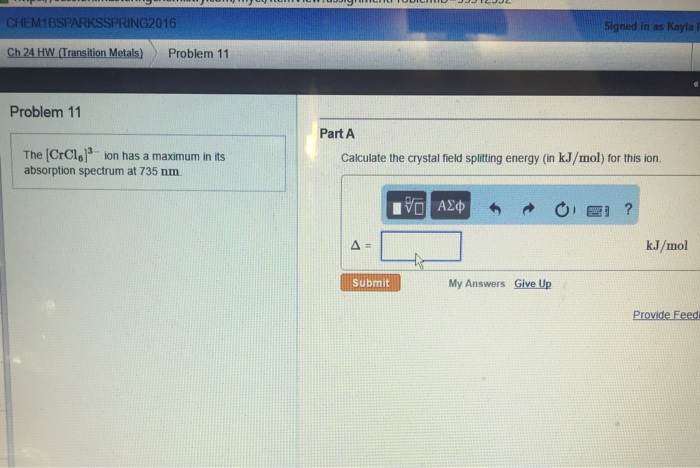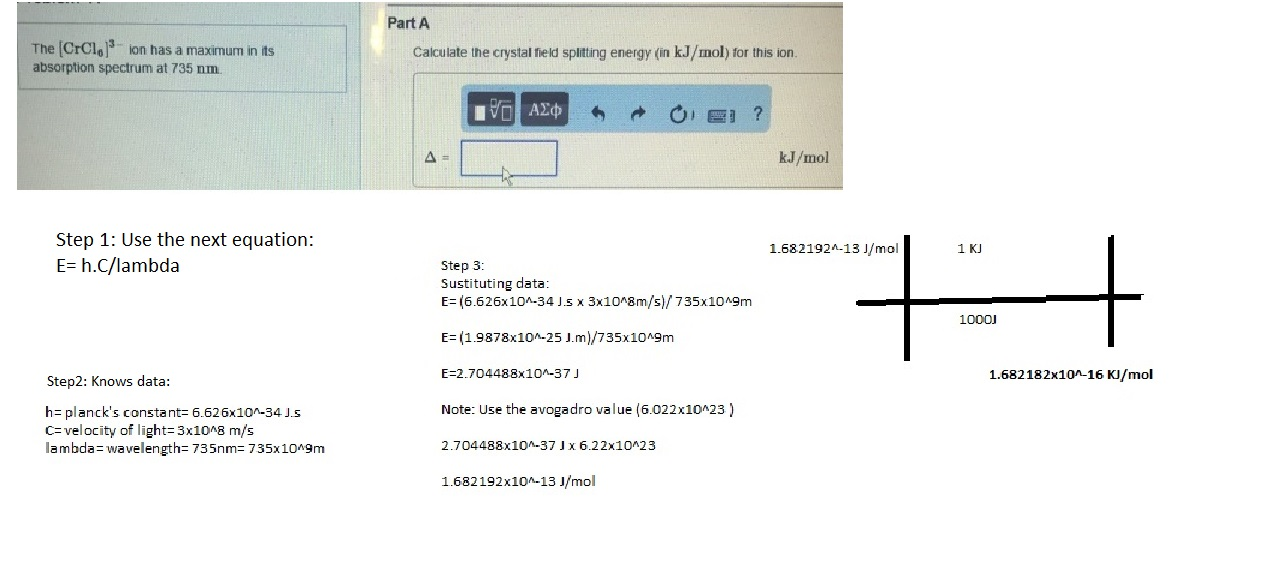#### Earn Coins

Coins can be redeemed for fabulous gifts.

Similar Homework Help Questions
• ### 620 nm Estimate the wavelength of maximum absorption for the octahedral ion trioxalatochromate(III) from the fact...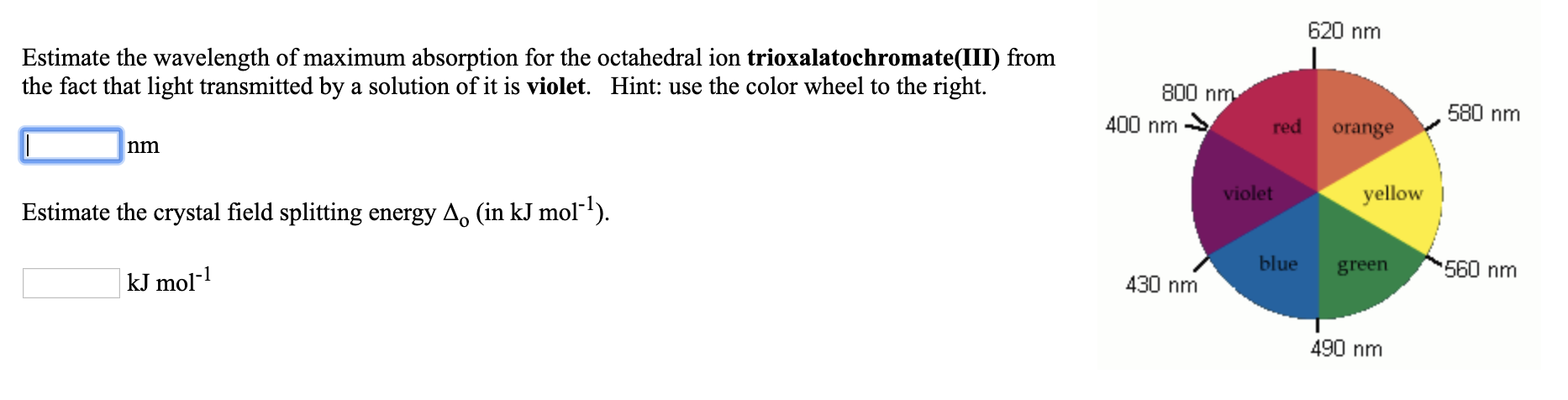620 nm Estimate the wavelength of maximum absorption for the octahedral ion trioxalatochromate(III) from the fact that light transmitted by a solution of it is violet. Hint: use the color wheel to the right. 800 nm 400 nm → red orange 580 nm violet yellow Estimate the crystal field splitting energy A. (in kJ mol-?). blue green kJ mol-1 560 nm 430 nm 490 nm

• ### A ?1 octahedral complex is found to absorb visible light, with the absorption maximum occurring at 499 nm . Calculate the crystal-field splitting energy, Δ , in kJ/mol.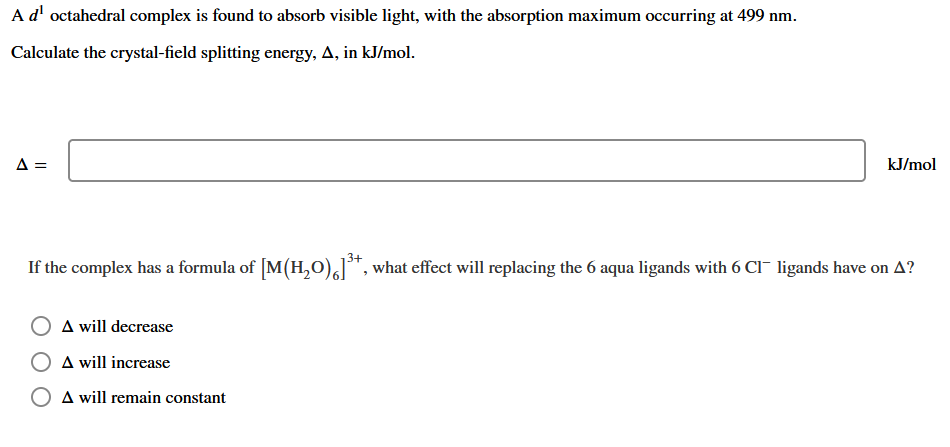A ?1 octahedral complex is found to absorb visible light, with the absorption maximum occurring at 499 nmCalculate the crystal-field splitting energy, Δin kJ/mol.

• ### CE IZOLIUM The (RhC16]has its maximum absorbance at 495 nm. 1. Calculate the crystal field splitting...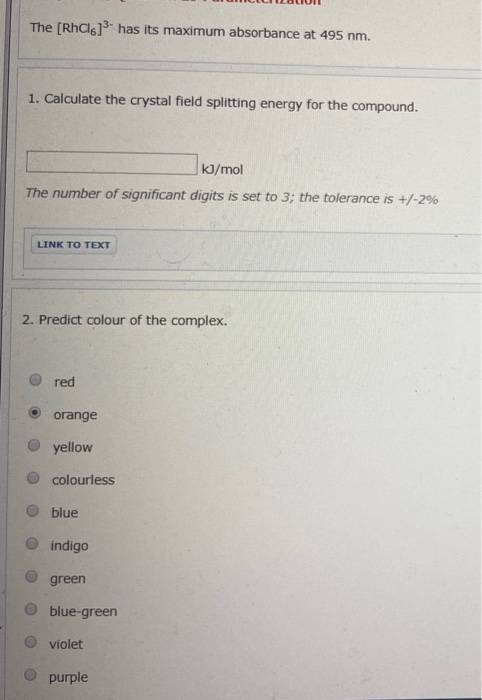CE IZOLIUM The (RhC16]has its maximum absorbance at 495 nm. 1. Calculate the crystal field splitting energy for the compound. kJ/mol The number of significant digits is set to 3; the tolerance is +/-2% LINK TO TEXT 2. Predict colour of the complex. red orange yellow O colourless O blue indigo O green blue-green violet O purple

• ### Estimate the wavelength of maximum absorption for the octahedral ion hexaamminecobalt(lII) from right the fact that...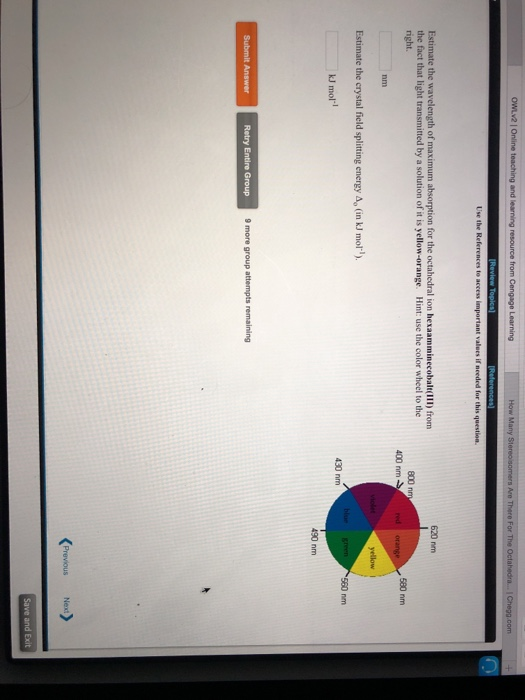Estimate the wavelength of maximum absorption for the octahedral ion hexaamminecobalt(lII) from right the fact that light transmitted by a solution of it is yellow-orange. Hint: use the color wheel to the 620 nm 800 nm 400 nm 580 nm nm Estimate the crystal field splitting energy Ap (in kJ mol) kJ mol-l 430 nm 490 nm Retry Entire Group 9 more group attempts remaining

• ### A solution containing [Fe(CN)6]3" has its maximum absorbance at 305 nm. Calculate the crystal field splitting...

A solution containing [Fe(CN)6]3" has its maximum absorbance at 305 nm. Calculate the crystal field splitting energy for the compound and predict its colour

• ### The extent of crystal field splitting is often determined from spectra. Given the wavelength (7.) of...The extent of crystal field splitting is often determined from spectra. Given the wavelength (7.) of maximum absorption, 2. = 295 nm, find the crystal field splitting energy (A), in kJ/mol, for the complex ion (Rh(NH3).13+. kJ/mol

• ### Estimate the crystal field stabilization energy for the octahedral ion hexacyanocobaltate(III), if the wavelength of maximum...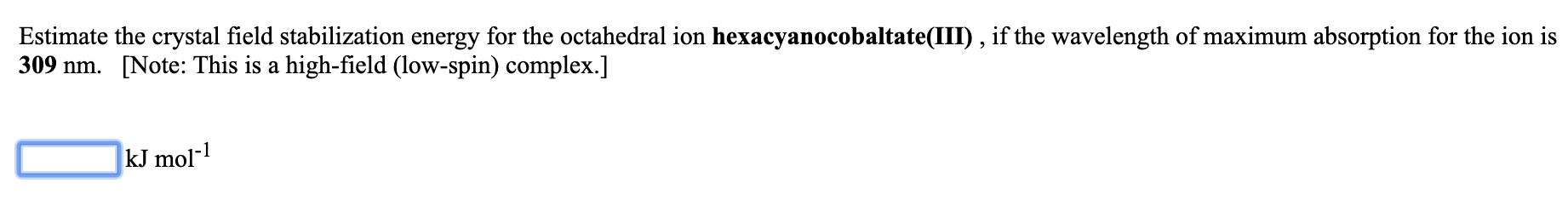Estimate the crystal field stabilization energy for the octahedral ion hexacyanocobaltate(III), if the wavelength of maximum absorption for the ion is 309 nm. [Note: This is a high-field (low-spin) complex.] kJ mol-1

• ### Estimate the crystal field stabilization energy for the octahedral ion hexafluorocobaltate(III), if the wavelength of maximum...

Estimate the crystal field stabilization energy for the octahedral ion hexafluorocobaltate(III), if the wavelength of maximum absorption for the ion is 710 nm.   [Note: This is a weak-field (high-spin) complex.] _____ kJ mol-1

• ### Ad octahedral complex is found to absorb visible light, with the absorption maximum occurring at 515...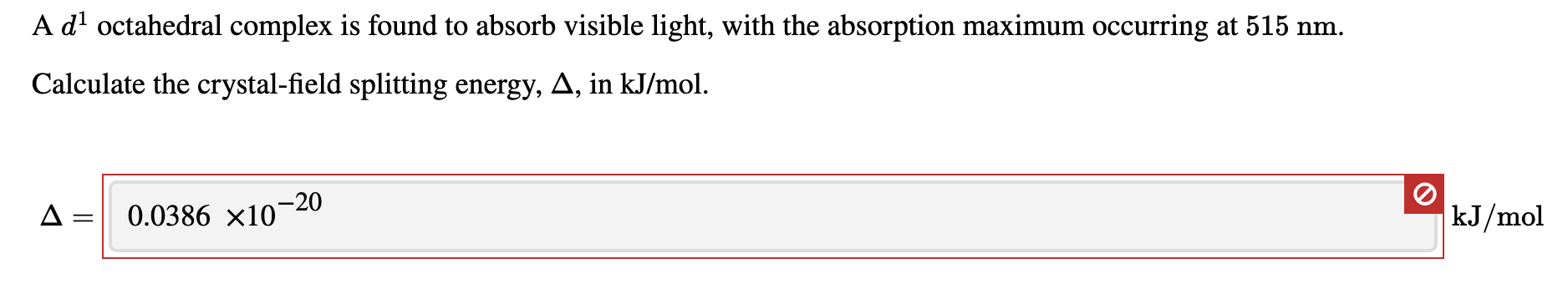Ad octahedral complex is found to absorb visible light, with the absorption maximum occurring at 515 nm. Calculate the crystal-field splitting energy, A, in kJ/mol. A = 0.0386 x10-20 kJ/mol

• ### A dl octahedral complex is found to absorb visible light, with the absorption maximum occurring at...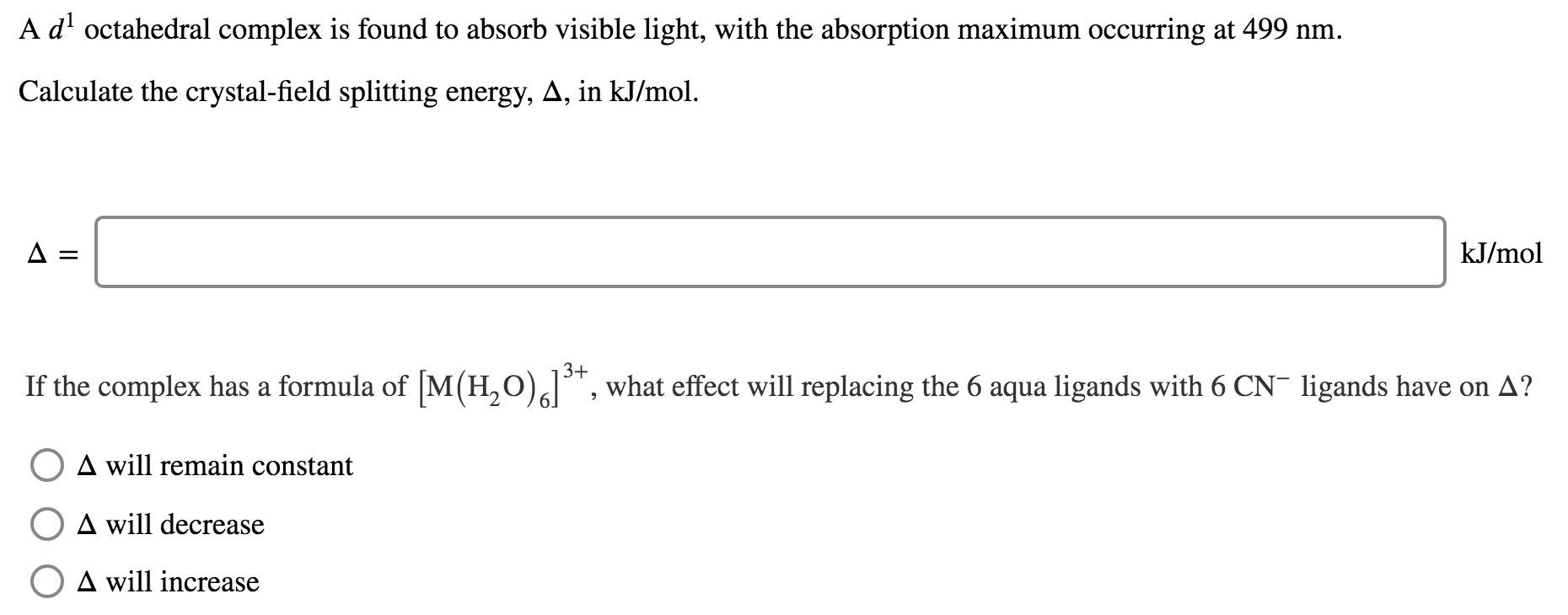A dl octahedral complex is found to absorb visible light, with the absorption maximum occurring at 499 nm. Calculate the crystal-field splitting energy, A, in kJ/mol. A= kJ/mol If the complex has a formula of [M(H20)alt, what effect will replacing the 6 aqua ligands with 6 CN- ligands have on A? O A will remain constant A will decrease O A will increase